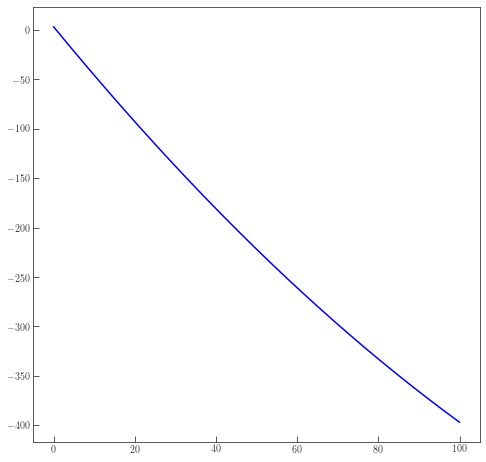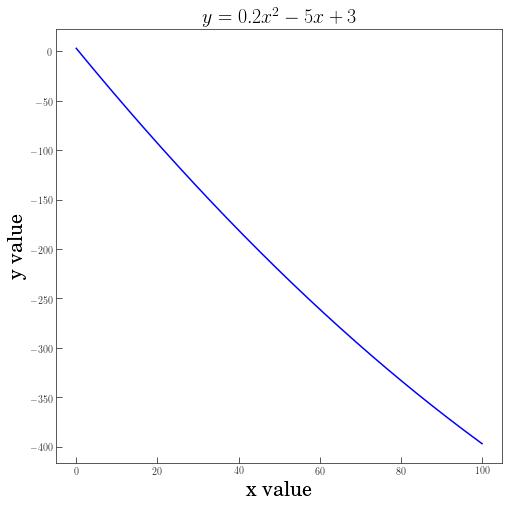# Week 5 - Numpy & Matplotlib¶

• Numpy
• Matplotlib

## Numpy - Numerical Python¶

From their website (http://www.numpy.org/):

NumPy is the fundamental package for scientific computing with Python.

• a powerful N-dimensional array object
• tools for integrating C/C++ and Fortran code
• useful linear algebra, Fourier transform, and random number capabilities

You can import "numpy" as

In :
import numpy as np


## Numpy arrays¶

In standard Python, data is stored as lists, and multidimensional data as lists of lists. In numpy, however, we can now work with arrays. To get these arrays, we can use np.asarray to convert a list into an array. Below we take a quick look at how a list behaves differently from an array.

In :
# We first create an array x
start = 1
stop  = 11
step  = 1

x = np.arange(start, stop, step)

print(x)

[ 1  2  3  4  5  6  7  8  9 10]


We can also manipulate the array. For example, we can:

• Multiply by two:
In :
x * 2

Out:
array([ 2,  4,  6,  8, 10, 12, 14, 16, 18, 20])
• Take the square of all the values in the array:
In :
x ** 2

Out:
array([  1,   4,   9,  16,  25,  36,  49,  64,  81, 100])
• Or even do some math on it:
In :
(x**2) + (5*x) + (x / 3)

Out:
array([  6.33333333,  14.66666667,  25.        ,  37.33333333,
51.66666667,  68.        ,  86.33333333, 106.66666667,
129.        , 153.33333333])

If we want to set up an array in numpy, we can use range to make a list and then convert it to an array, but we can also just create an array directly in numpy. np.arange will do this with integers, and np.linspace will do this with floats, and allows for non-integer steps.

In :
print(np.arange(10))

print(np.linspace(1,10,10))

[0 1 2 3 4 5 6 7 8 9]
[ 1.  2.  3.  4.  5.  6.  7.  8.  9. 10.]


Last week we had to use a function or a loop to carry out math on a list. However with numpy we can do this a lot simpler by making sure we're working with an array, and carrying out the mathematical operations on that array.

In :
x=np.arange(10)
print(x)

print(x**2)

[0 1 2 3 4 5 6 7 8 9]
[ 0  1  4  9 16 25 36 49 64 81]


In numpy, we also have more options for quickly (and without much code) examining the contents of an array. One of the most helpful tools for this is np.where. np.where uses a conditional statement on the array and returns an array that contains indices of all the values that were true for the conditional statement. We can then call the original array and use the new array to get all the values that were true for the conditional statement.

There are also functions like max and min that will give the maximum and minimum, respectively.

In :
# Defining starting and ending values of the array, as well as the number of elements in the array.
start = 0
stop  = 100
n_elements = 201

x = np.linspace(start, stop, n_elements)

print(x)

[  0.    0.5   1.    1.5   2.    2.5   3.    3.5   4.    4.5   5.    5.5
6.    6.5   7.    7.5   8.    8.5   9.    9.5  10.   10.5  11.   11.5
12.   12.5  13.   13.5  14.   14.5  15.   15.5  16.   16.5  17.   17.5
18.   18.5  19.   19.5  20.   20.5  21.   21.5  22.   22.5  23.   23.5
24.   24.5  25.   25.5  26.   26.5  27.   27.5  28.   28.5  29.   29.5
30.   30.5  31.   31.5  32.   32.5  33.   33.5  34.   34.5  35.   35.5
36.   36.5  37.   37.5  38.   38.5  39.   39.5  40.   40.5  41.   41.5
42.   42.5  43.   43.5  44.   44.5  45.   45.5  46.   46.5  47.   47.5
48.   48.5  49.   49.5  50.   50.5  51.   51.5  52.   52.5  53.   53.5
54.   54.5  55.   55.5  56.   56.5  57.   57.5  58.   58.5  59.   59.5
60.   60.5  61.   61.5  62.   62.5  63.   63.5  64.   64.5  65.   65.5
66.   66.5  67.   67.5  68.   68.5  69.   69.5  70.   70.5  71.   71.5
72.   72.5  73.   73.5  74.   74.5  75.   75.5  76.   76.5  77.   77.5
78.   78.5  79.   79.5  80.   80.5  81.   81.5  82.   82.5  83.   83.5
84.   84.5  85.   85.5  86.   86.5  87.   87.5  88.   88.5  89.   89.5
90.   90.5  91.   91.5  92.   92.5  93.   93.5  94.   94.5  95.   95.5
96.   96.5  97.   97.5  98.   98.5  99.   99.5 100. ]


And we can select only those values that are divisible by 5:

In :
# This function returns the indices that match the criteria of x % 5 == 0:
x_5 = np.where(x%5 == 0)

print(x_5)

# And one can use those indices to *only* select those values:
print(x[x_5])

(array([  0,  10,  20,  30,  40,  50,  60,  70,  80,  90, 100, 110, 120,
130, 140, 150, 160, 170, 180, 190, 200]),)
[  0.   5.  10.  15.  20.  25.  30.  35.  40.  45.  50.  55.  60.  65.
70.  75.  80.  85.  90.  95. 100.]


Or similarly:

In :
x[x%5 == 0]

Out:
array([  0.,   5.,  10.,  15.,  20.,  25.,  30.,  35.,  40.,  45.,  50.,
55.,  60.,  65.,  70.,  75.,  80.,  85.,  90.,  95., 100.])

And you can find the max and min values of the array:

In :
print('The minimum of x is {0}'.format(x.min()))

print('The maximum of x is {0}'.format(x.max()))

The minimum of x is 0.0
The maximum of x is 100.0


Numpy also provides some tools for loading and saving data, loadtxt and savetxt. Here I'm using a function called transpose so that instead of each array being a row, they each get treated as a column.

When we load the information again, it's now a 2D array. We can select parts of those arrays just as we could for 1D arrays.

In :
start  = 0
stop   = 100
n_elem = 501

x = np.linspace(start, stop, n_elem)

# We can now create another array from x:
y = (.1*x)**2 - (5*x) + 3

# And finally, we can dump x and y to a file:
np.savetxt('myfile.txt', np.transpose([x,y]))

# We can also load the data from myfile.txt and display it:
print('2D-array from file myfile.txt:\n\n', data, '\n')

# You can also select certain elements of the 2D-array
print('Selecting certain elements from data:\n\n', data[:3,:], '\n')

2D-array from file myfile.txt:

[[ 0.000000e+00  3.000000e+00]
[ 2.000000e-01  2.000400e+00]
[ 4.000000e-01  1.001600e+00]
...
[ 9.960000e+01 -3.957984e+02]
[ 9.980000e+01 -3.963996e+02]
[ 1.000000e+02 -3.970000e+02]]

Selecting certain elements from data:

[[0.     3.    ]
[0.2    2.0004]
[0.4    1.0016]]



## Matplotlib¶

Matplotlib is a Python 2D plotting library which

• produces publication quality figures in a variety of hardcopy formats and interactive environments across platforms
• Quick way to visualize data from Python
• Main plotting utility in Python

From their website (http://matplotlib.org/):

Matplotlib is a Python 2D plotting library which produces publication quality figures in a variety of hardcopy formats and interactive environments across platforms. Matplotlib can be used in Python scripts, the Python and IPython shell, the jupyter notebook, web application servers, and four graphical user interface toolkits.

A great starting point to figuring out how to make a particular figure is to start from the Matplotlib gallery and look for what you want to make.

In :
## Importing modules
%matplotlib inline

# Importing LaTeX
from matplotlib import rc
rc('text', usetex=True)

# Importing matplotlib and other modules
import matplotlib.pyplot as plt
import numpy as np


We can now load in the data from myfile.txt

In :
data = np.loadtxt('myfile.txt')


The simplest figure is to simply make a plot. We can have multiple figures, but for now, just one. The plt.plot function will connect the points, but if we want a scatter plot, then plt.scatter will work.

In :
plt.figure(1, figsize=(8,8))
plt.plot(data[:,0],data[:,1])
plt.show()You can also pass the *data.T value instead:

In :
plt.figure(1, figsize=(8,8))
plt.plot(*data.T)
plt.show()We can take that same figure and add on the needed labels and titles.

In :
# Creating figure
plt.figure(figsize=(8,8))
plt.plot(*data.T)
plt.title(r'$y = 0.2x^{2} - 5x + 3$', fontsize=20)
plt.xlabel('x value', fontsize=20)
plt.ylabel('y value', fontsize=20)
plt.show()There's a large number of options available for plotting, so try using the initial code below, combined with the information here to try out a few of the following things: changing the line width, changing the line color

In :
plt.figure(figsize=(8,8))
plt.plot(data[:,0],data[:,1])
plt.title(r'$y = 0.2x^{2} - 5x + 3$', fontsize=20)
plt.xlabel('x value', fontsize=20)
plt.ylabel('y value', fontsize=20)
plt.show()